# class TPrincipal: public TNamed



>

# Principal Components Analysis (PCA)

The current implementation is based on the LINTRA package from CERNLIB by R. Brun, H. Hansroul, and J. Kubler. The class has been implemented by Christian Holm Christensen in August 2000.

## Introduction

In many applications of various fields of research, the treatment of large amounts of data requires powerful techniques capable of rapid data reduction and analysis. Usually, the quantities most conveniently measured by the experimentalist, are not necessarily the most significant for classification and analysis of the data. It is then useful to have a way of selecting an optimal set of variables necessary for the recognition process and reducing the dimensionality of the problem, resulting in an easier classification procedure.

This paper describes the implementation of one such method of feature selection, namely the principal components analysis. This multidimensional technique is well known in the field of pattern recognition and and its use in Particle Physics has been documented elsewhere (cf. H. Wind, Function Parameterization, CERN 72-21).

## Overview

Suppose we have prototypes which are trajectories of particles, passing through a spectrometer. If one measures the passage of the particle at say 8 fixed planes, the trajectory is described by an 8-component vector: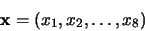in 8-dimensional pattern space.

One proceeds by generating a a representative tracks sample and building up the covariance matrix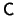. Its eigenvectors and eigenvalues are computed by standard methods, and thus a new basis is obtained for the original 8-dimensional space the expansion of the prototypes,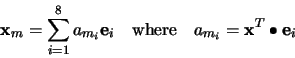allows the study of the behavior of the coefficients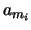for all the tracks of the sample. The eigenvectors which are insignificant for the trajectory description in the expansion will have their corresponding coefficientsclose to zero for all the prototypes.

On one hand, a reduction of the dimensionality is then obtained by omitting these least significant vectors in the subsequent analysis.

On the other hand, in the analysis of real data, these least significant variables(?) can be used for the pattern recognition problem of extracting the valid combinations of coordinates describing a true trajectory from the set of all possible wrong combinations.

The program described here performs this principal components analysis on a sample of data provided by the user. It computes the covariance matrix, its eigenvalues ands corresponding eigenvectors and exhibits the behavior of the principal components (), thus providing to the user all the means of understanding their data.

## Principal Components Method

Let's consider a sample of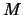prototypes each being characterized by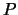variables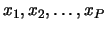. Each prototype is a point, or a column vector, in a-dimensional pattern space.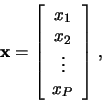(1)

where each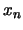represents the particular value associated with the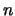-dimension.

Thosevariables are the quantities accessible to the experimentalist, but are not necessarily the most significant for the classification purpose.

The Principal Components Method consists of applying a linear transformation to the original variables. This transformation is described by an orthogonal matrix and is equivalent to a rotation of the original pattern space into a new set of coordinate vectors, which hopefully provide easier feature identification and dimensionality reduction.

Let's define the covariance matrix: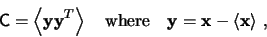(2)

and the brackets indicate mean value over the sample ofprototypes.

This matrixis real, positive definite, symmetric, and will have all its eigenvalues greater then zero. It will now be show that among the family of all the complete orthonormal bases of the pattern space, the base formed by the eigenvectors of the covariance matrix and belonging to the largest eigenvalues, corresponds to the most significant features of the description of the original prototypes.

let the prototypes be expanded on into a set of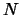basis vectors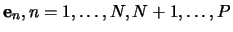,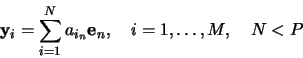(3)

The best' feature coordinates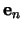, spanning a feature space, will be obtained by minimizing the error due to this truncated expansion, i.e.,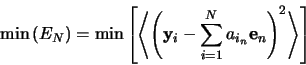(4)

with the conditions: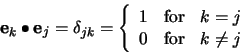(5)

Multiplying (3) by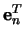using (5), we get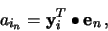(6)

so the error becomes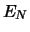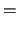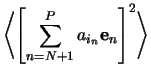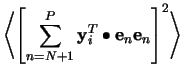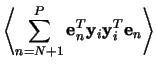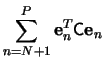(7)

The minimization of the sum in (7) is obtained when each term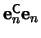is minimum, sinceis positive definite. By the method of Lagrange multipliers, and the condition (5), we get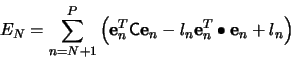(8)

The minimum condition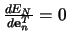leads to the equation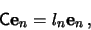(9)

which shows thatis an eigenvector of the covariance matrixwith eigenvalue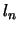. The estimated minimum error is then given by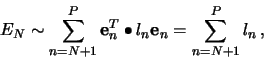(10)

where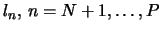are the eigenvalues associated with the omitted eigenvectors in the expansion (3). Thus, by choosing thelargest eigenvalues, and their associated eigenvectors, the erroris minimized.

The transformation matrix to go from the pattern space to the feature space consists of the ordered eigenvectors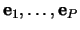for its columns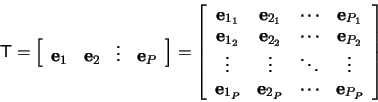(11)

This is an orthogonal transformation, or rotation, of the pattern space and feature selection results in ignoring certain coordinates in the transformed space.

Christian Holm
August 2000, CERN

## Function Members (Methods)

public:
 virtual ~TPrincipal() void TObject::AbstractMethod(const char* method) const virtual void AddRow(const Double_t* x) virtual void TObject::AppendPad(Option_t* option = "") virtual void Browse(TBrowser* b) static TClass* Class() virtual const char* TObject::ClassName() const virtual void Clear(Option_t* option = "") virtual TObject* TNamed::Clone(const char* newname = "") const virtual Int_t TNamed::Compare(const TObject* obj) const virtual void TNamed::Copy(TObject& named) const virtual void TObject::Delete(Option_t* option = "")MENU virtual Int_t TObject::DistancetoPrimitive(Int_t px, Int_t py) virtual void TObject::Draw(Option_t* option = "") virtual void TObject::DrawClass() constMENU virtual TObject* TObject::DrawClone(Option_t* option = "") constMENU virtual void TObject::Dump() constMENU virtual void TObject::Error(const char* method, const char* msgfmt) const virtual void TObject::Execute(const char* method, const char* params, Int_t* error = 0) virtual void TObject::Execute(TMethod* method, TObjArray* params, Int_t* error = 0) virtual void TObject::ExecuteEvent(Int_t event, Int_t px, Int_t py) virtual void TObject::Fatal(const char* method, const char* msgfmt) const virtual void TNamed::FillBuffer(char*& buffer) virtual TObject* TObject::FindObject(const char* name) const virtual TObject* TObject::FindObject(const TObject* obj) const const TMatrixD* GetCovarianceMatrix() const virtual Option_t* TObject::GetDrawOption() const static Long_t TObject::GetDtorOnly() const TVectorD* GetEigenValues() const const TMatrixD* GetEigenVectors() const TList* GetHistograms() const virtual const char* TObject::GetIconName() const const TVectorD* GetMeanValues() const virtual const char* TNamed::GetName() const virtual char* TObject::GetObjectInfo(Int_t px, Int_t py) const static Bool_t TObject::GetObjectStat() virtual Option_t* TObject::GetOption() const const Double_t* GetRow(Int_t row) const TVectorD* GetSigmas() const virtual const char* TNamed::GetTitle() const virtual UInt_t TObject::GetUniqueID() const const TVectorD* GetUserData() const virtual Bool_t TObject::HandleTimer(TTimer* timer) virtual ULong_t TNamed::Hash() const virtual void TObject::Info(const char* method, const char* msgfmt) const virtual Bool_t TObject::InheritsFrom(const char* classname) const virtual Bool_t TObject::InheritsFrom(const TClass* cl) const virtual void TObject::Inspect() constMENU void TObject::InvertBit(UInt_t f) virtual TClass* IsA() const virtual Bool_t TObject::IsEqual(const TObject* obj) const virtual Bool_t IsFolder() const Bool_t TObject::IsOnHeap() const virtual Bool_t TNamed::IsSortable() const Bool_t TObject::IsZombie() const virtual void TNamed::ls(Option_t* option = "") const virtual void MakeCode(const char* filename = "pca", Option_t* option = "")MENU virtual void MakeHistograms(const char* name = "pca", Option_t* option = "epsdx")MENU virtual void MakeMethods(const char* classname = "PCA", Option_t* option = "")MENU virtual void MakePrincipals()MENU void TObject::MayNotUse(const char* method) const virtual Bool_t TObject::Notify() void TObject::Obsolete(const char* method, const char* asOfVers, const char* removedFromVers) const void TObject::operator delete(void* ptr) void TObject::operator delete(void* ptr, void* vp) void TObject::operator delete[](void* ptr) void TObject::operator delete[](void* ptr, void* vp) void* TObject::operator new(size_t sz) void* TObject::operator new(size_t sz, void* vp) void* TObject::operator new[](size_t sz) void* TObject::operator new[](size_t sz, void* vp) virtual void P2X(const Double_t* p, Double_t* x, Int_t nTest) virtual void TObject::Paint(Option_t* option = "") virtual void TObject::Pop() virtual void Print(Option_t* opt = "MSE") constMENU virtual Int_t TObject::Read(const char* name) virtual void TObject::RecursiveRemove(TObject* obj) void TObject::ResetBit(UInt_t f) virtual void TObject::SaveAs(const char* filename = "", Option_t* option = "") constMENU virtual void TObject::SavePrimitive(ostream& out, Option_t* option = "") void TObject::SetBit(UInt_t f) void TObject::SetBit(UInt_t f, Bool_t set) virtual void TObject::SetDrawOption(Option_t* option = "")MENU static void TObject::SetDtorOnly(void* obj) virtual void TNamed::SetName(const char* name)MENU virtual void TNamed::SetNameTitle(const char* name, const char* title) static void TObject::SetObjectStat(Bool_t stat) virtual void TNamed::SetTitle(const char* title = "")MENU virtual void TObject::SetUniqueID(UInt_t uid) virtual void ShowMembers(TMemberInspector& insp) const virtual Int_t TNamed::Sizeof() const virtual void Streamer(TBuffer&) void StreamerNVirtual(TBuffer& ClassDef_StreamerNVirtual_b) virtual void SumOfSquareResiduals(const Double_t* x, Double_t* s) virtual void TObject::SysError(const char* method, const char* msgfmt) const void Test(Option_t* option = "")MENU Bool_t TObject::TestBit(UInt_t f) const Int_t TObject::TestBits(UInt_t f) const TPrincipal() TPrincipal(Int_t nVariables, Option_t* opt = "ND") virtual void TObject::UseCurrentStyle() virtual void TObject::Warning(const char* method, const char* msgfmt) const virtual Int_t TObject::Write(const char* name = 0, Int_t option = 0, Int_t bufsize = 0) virtual Int_t TObject::Write(const char* name = 0, Int_t option = 0, Int_t bufsize = 0) const virtual void X2P(const Double_t* x, Double_t* p)
protected:
 virtual void TObject::DoError(int level, const char* location, const char* fmt, va_list va) const void MakeNormalised() void MakeRealCode(const char* filename, const char* prefix, Option_t* option = "") void TObject::MakeZombie() TPrincipal& operator=(const TPrincipal&) TPrincipal(const TPrincipal&)

## Data Members

public:
 static TObject::(anonymous) TObject::kBitMask static TObject::EStatusBits TObject::kCanDelete static TObject::EStatusBits TObject::kCannotPick static TObject::EStatusBits TObject::kHasUUID static TObject::EStatusBits TObject::kInvalidObject static TObject::(anonymous) TObject::kIsOnHeap static TObject::EStatusBits TObject::kIsReferenced static TObject::EStatusBits TObject::kMustCleanup static TObject::EStatusBits TObject::kNoContextMenu static TObject::(anonymous) TObject::kNotDeleted static TObject::EStatusBits TObject::kObjInCanvas static TObject::(anonymous) TObject::kOverwrite static TObject::(anonymous) TObject::kSingleKey static TObject::(anonymous) TObject::kWriteDelete static TObject::(anonymous) TObject::kZombie
protected:
 TMatrixD fCovarianceMatrix Covariance matrix TVectorD fEigenValues Eigenvalue vector of trans TMatrixD fEigenVectors Eigenvector matrix of trans TList* fHistograms List of histograms Bool_t fIsNormalised Normalize matrix? TVectorD fMeanValues Mean value over all data points TString TNamed::fName object identifier Int_t fNumberOfDataPoints Number of data points Int_t fNumberOfVariables Number of variables TVectorD fOffDiagonal elements of the tridiagonal TVectorD fSigmas vector of sigmas Bool_t fStoreData Should we store input data? TString TNamed::fTitle object title Double_t fTrace Trace of covarience matrix TVectorD fUserData Vector of original data points

## Class Charts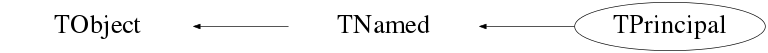## Function documentation

 Empty CTOR, Do not use.

TPrincipal(Int_t nVariables, Option_t* opt = "ND")
 Ctor. Argument is number of variables in the sample of data
Options are:
N       Normalize the covariance matrix (default)
D       Store input data (default)

The created object is  named "principal" by default.

TPrincipal(const TPrincipal& )
copy constructor

TPrincipal& operator=(const TPrincipal& )
assignement operator


 destructor

/*
>
Add a data point and update the covariance matrix. The input
array must be fNumberOfVariables long.

The Covariance matrix and mean values of the input data is caculated on the fly by the following equations: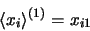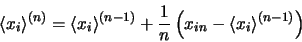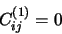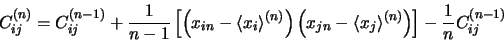since this is a really fast method, with no rounding errors (please refer to CERN 72-21 pp. 54-106).

The data is stored internally in a TVectorD, in the following way: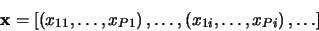Withas defined in the class description.
  */


void Browse(TBrowser* b)
 Browse the TPrincipal object in the TBrowser.

void Clear(Option_t* option = "")
 Clear the data in Object. Notice, that's not possible to change
the dimension of the original data.

const Double_t * GetRow(Int_t row)
 Return a row of the user supplied data.
If row is out of bounds, 0 is returned.
It's up to the user to delete the returned array.
Row 0 is the first row;

void MakeCode(const char* filename = "pca", Option_t* option = "")
 Generates the file <filename>, with .C appended if it does
argument doesn't end in .cxx or .C.

The file contains the implementation of two functions

void X2P(Double_t *x, Double *p)
void P2X(Double_t *p, Double *x, Int_t nTest)

which does the same as  TPrincipal::X2P and TPrincipal::P2X
respectively. Please refer to these methods.

Further, the static variables:

Int_t    gNVariables
Double_t gEigenValues[]
Double_t gEigenVectors[]
Double_t gMeanValues[]
Double_t gSigmaValues[]

are initialized. The only ROOT header file needed is Rtypes.h

See TPrincipal::MakeRealCode for a list of options

void MakeHistograms(const char* name = "pca", Option_t* option = "epsdx")
 Make histograms of the result of the analysis.
The option string say which histograms to create
X         Histogram original data
P         Histogram principal components corresponding to
original data
D         Histogram the difference between the original data
and the projection of principal unto a lower
dimensional subspace (2D histograms)
E         Histogram the eigenvalues
S         Histogram the square of the residues
(see TPrincipal::SumOfSquareResidues)
The histograms will be named <name>_<type><number>, where <name>
is the first argument, <type> is one of X,P,D,E,S, and <number>
is the variable.

void MakeNormalised()
 PRIVATE METHOD: Normalize the covariance matrix

void MakeMethods(const char* classname = "PCA", Option_t* option = "")
 Generate the file <classname>PCA.cxx which contains the
implementation of two methods:

void <classname>::X2P(Double_t *x, Double *p)
void <classname>::P2X(Double_t *p, Double *x, Int_t nTest)

which does the same as  TPrincipal::X2P and TPrincipal::P2X
respectivly. Please refer to these methods.

Further, the public static members:

Int_t    <classname>::fgNVariables
Double_t <classname>::fgEigenValues[]
Double_t <classname>::fgEigenVectors[]
Double_t <classname>::fgMeanValues[]
Double_t <classname>::fgSigmaValues[]

are initialized, and assumed to exist. The class declaration is
assumed to be in <classname>.h and assumed to be provided by the
user.

See TPrincipal::MakeRealCode for a list of options

The minimal class definition is:

class <classname> {
public:
static Int_t    fgNVariables;
static Double_t fgEigenVectors[];
static Double_t fgEigenValues[];
static Double_t fgMeanValues[];
static Double_t fgSigmaValues[];

void X2P(Double_t *x, Double_t *p);
void P2X(Double_t *p, Double_t *x, Int_t nTest);
};

Whether the methods <classname>::X2P and <classname>::P2X should
be static or not, is up to the user.

void MakePrincipals()
 Perform the principal components analysis.
This is done in several stages in the TMatrix::EigenVectors method:
* Transform the covariance matrix into a tridiagonal matrix.
* Find the eigenvalues and vectors of the tridiagonal matrix.

void MakeRealCode(const char* filename, const char* prefix, Option_t* option = "")
 PRIVATE METHOD:
This is the method that actually generates the code for the
transformations to and from feature space and pattern space
It's called by TPrincipal::MakeCode and TPrincipal::MakeMethods.

The options are: NONE so far

void P2X(const Double_t* p, Double_t* x, Int_t nTest)
 Calculate x as a function of nTest of the most significant
principal components p, and return it in x.
It's the users responsibility to make sure that both x and p are
of the right size (i.e., memory must be allocated for x).

void Print(Option_t* opt = "MSE") const
 Print the statistics
Options are
M            Print mean values of original data
S            Print sigma values of original data
E            Print eigenvalues of covariance matrix
V            Print eigenvectors of covariance matrix
Default is MSE

void SumOfSquareResiduals(const Double_t* x, Double_t* s)
 PRIVATE METHOD:


/* > Calculates the sum of the square residuals, that is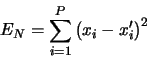where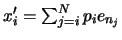,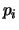is the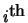component of the principal vector, corresponding to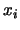, the original data; I.e., the square distance to the space spanned byeigenvectors.
   */


void Test(Option_t* option = "")
 Test the PCA, bye calculating the sum square of residuals
(see method SumOfSquareResiduals), and display the histogram

void X2P(const Double_t* x, Double_t* p)
 Calculate the principal components from the original data vector
x, and return it in p.
It's the users responsibility to make sure that both x and p are
of the right size (i.e., memory must be allocated for p).

const TMatrixD * GetCovarianceMatrix() const
{return &fCovarianceMatrix;}
const TVectorD * GetEigenValues() const
{return &fEigenValues;}
const TMatrixD * GetEigenVectors() const
{return &fEigenVectors;}
TList * GetHistograms() const
{return fHistograms;}
const TVectorD * GetMeanValues() const
{return &fMeanValues;}
const TVectorD * GetSigmas() const
{return &fSigmas;}
const TVectorD * GetUserData() const
{return &fUserData;}
Bool_t IsFolder() const
{ return kTRUE;}`